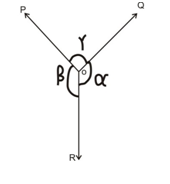Lami’s theorem is one such equation which relates the extent of three coplanar, non-collinear forces and concurrent forces. It is crucial to mention that the object always remains in static position. This theorem also states that in case there are three different forces which acts at a given point known are in equilibrium, then every single force will be proportional to the sine of the angle among two other forces.

Let’s understand the concept of Lami’s Theorem through a graphical representation.Suppose, three different forces are P, Q and R and they are acting on a particle or rigid body, consequently forming angles γ, α and β.

Let’s now learn the mathematical representation of Lami’s Theorem –

p/sinα = Q/sin β =  S/ sin γ

Links of Next Mechanical Engineering Topics:-### Customer Reviews

My Homework Help
Rated 5.0 out of 5 based on 510 customer reviews at